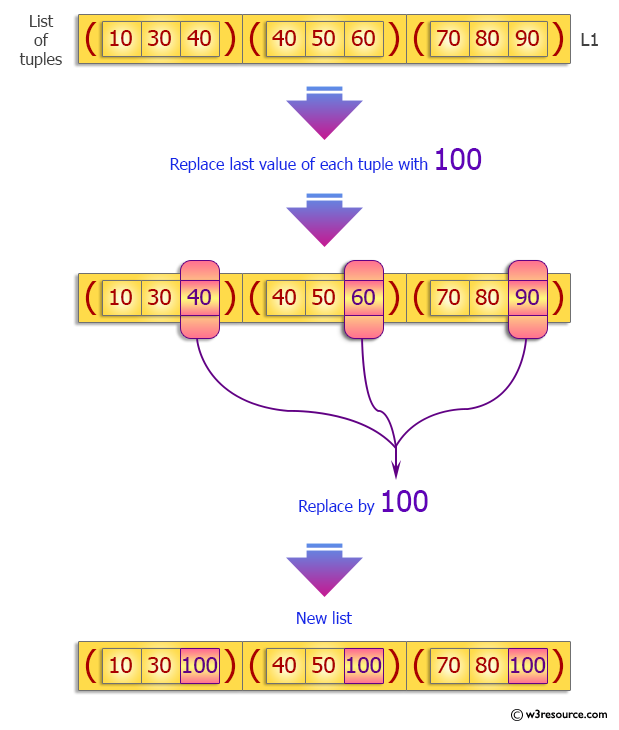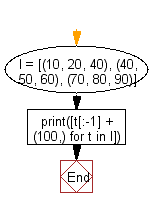﻿ Python: Replace last value of tuples in a list - w3resource# Python Exercise: Replace last value of tuples in a list

## Python tuple: Exercise-21 with Solution

Write a Python program to replace last value of tuples in a list.

Sample Solution:-

Python Code:

``````l = [(10, 20, 40), (40, 50, 60), (70, 80, 90)]
print([t[:-1] + (100,) for t in l])
```
```

Sample Output:

```[(10, 20, 100), (40, 50, 100), (70, 80, 100)]
```

Pictorial Presentation:Flowchart:## Visualize Python code execution:

The following tool visualize what the computer is doing step-by-step as it executes the said program:

Python Code Editor:

Have another way to solve this solution? Contribute your code (and comments) through Disqus.

What is the difficulty level of this exercise?

Test your Programming skills with w3resource's quiz.

﻿

## Python: Tips of the Day

Decapitalizes the first letter of a string:

Example:

```def tips_decapitalize(s, upper_rest=False):
return s[:1].lower() + (s[1:].upper() if upper_rest else s[1:])
print(tips_decapitalize('PythonTips'))
print(tips_decapitalize('PythonTips', True))
```

Output:

```pythonTips
pYTHONTIPS
```